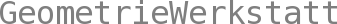Integrable Systems (and Infinite Dimensional Lie Algebras)

SS 2021  •

Prof Dr Christoph Bohle
Assistent: Jonas Ziefle

Integrable systems are differential or difference equations with extraordinarily large symmetry group. The course will focus on equations related to the Korteweg de Vries (KdV) equation and discrete counterparts. Originally a mathematical model for the soliton phenomenon discovered during a famous horse ride along a canal, equations of KdV type have now many applications and the underlying theory involves various mathematical disciplines.

A fundamental idea for understanding and solving KdV type equations is their interpretation as spectrum preserving deformations of underlying auxiliary linear operators - in the simplest case symmetric matrices.

This lecture is the continuation of the lecture called "Introduction to Integrable Systems (Classical Mechanics, Riemann Surfaces, and Spectral Theory)". This continuation will investigate integrable equations using sl(2,C)--loop algebras. In particular, we will study explcit solutions that can be described using the theory of hyperelliptic Riemann surfaces.

(Prerequisites: Basic knowledge of the material presented in Part I of the lecture called "Introduction to Integrable Systems (Classical Mechanics, Riemann Surfaces, and Spectral Theory)")

The lectures will be held online - in the same format as last semester. The exercise sessions in a hybrid format (partially online and - if possible - partially at the institute). Schedule for synchronous part:
 Lecture Tue.+Thu. 12.30-13.30 online Excercise ? ? +online.
Everything will be recorded. Further information as well as the course material and links to the lectures can be found in